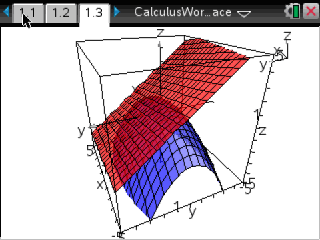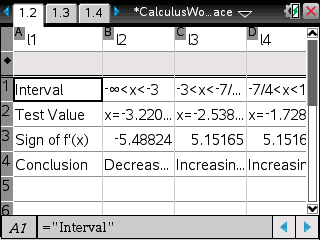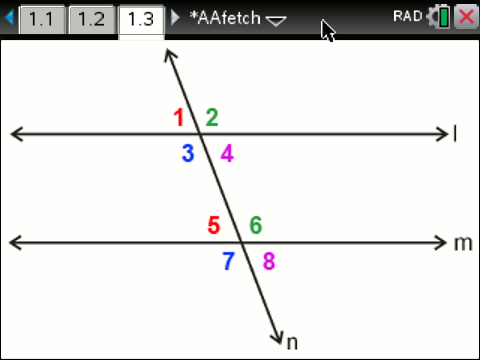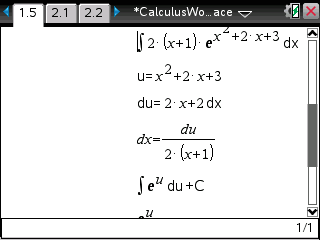Basics Archives Community Services ProgrammingHome :: Archives :: File Archives :: Math Equations Library 4.0 (CAS)Math Equations Library 4.0 (CAS)

FILE INFORMATION

mathlibrary.zip
 Filename mathlibrary.zip (Download) Title Math Equations Library 4.0 (CAS) Description This library contains formula sheets and programs for geometry, precalculus, calculus I-III, statistics, and linear algebra. It also contains a search engine for formulas. For example, AAFetch/fetch("trig identity") brings up a list of trig identities, and then you could type fetch("sum and difference identity"). You can navigate through it by entering fetch("algebra") or entering "geometry", "trigonometry", "statistics", "Calculus I", "Calculus II", "Calculus III", "linear algebra", or "differential equations". The readme file has more details on how the package's programs work. Author Rachel Bush (rachel.h.bush@gmail.com) Category TI-Nspire BASIC Math Programs File Size 3,155,224 bytes File Date and Time Thu May 27 15:34:38 2021 Documentation Included? Yes Source Code Included? Yes

SCREEN SHOTSREVIEWS

There are no reviews for this file.

LEAVE FEEDBACK

Questions, comments, and problems regarding the file itself should be sent directly to the author(s) listed above.

Report inappropriate or miscategorized file (requires an account; or you may email us directly)

 Bad Good 1  2  3  4  5  6  7  8  9  10

ARCHIVE CONTENTS

Archive Contents
Name Size
3.0 Release Notes.txt   2398
AAfetch.tns   632700
AAStatistics.tns   7260
corresponding.gif   6938
exteriorangle.gif   24444
multiregression.gif   16250
Programmingtools.tns   29009
Screenshot1.gif   14252
Screenshot2.gif   8769
Screenshot3.gif   4470
Screenshot4.gif   107810
mathlibrary/4.0 Release Notes.txt   3739
mathlibrary/corresponding.gif   6938
mathlibrary/exteriorangle.gif   24444
mathlibrary/multiregression.gif   16250
mathlibrary/MyLib/AACalculus.tns   10752
mathlibrary/MyLib/AACalculus_III.tns   6055
mathlibrary/MyLib/AAFetch.tns   1174667
mathlibrary/MyLib/AALinalg.tns   4634
mathlibrary/MyLib/AALinalg_Interpo.tns   4761
mathlibrary/MyLib/AALinalg_Matseq.tns   3245
mathlibrary/MyLib/AALinalg_Vectors.tns   8037
mathlibrary/MyLib/AAPrecalculus.tns   4704
mathlibrary/MyLib/AAStatistics.tns   7260
mathlibrary/MyLib/AAVectorAnalysis.tns   22611
mathlibrary/MyLib/Programmingtools.tns   29009
mathlibrary/Screenshot1.gif   14252
mathlibrary/Screenshot2.gif   8769
mathlibrary/Screenshot3.gif   4470
mathlibrary/Screenshot4.gif   107810
mathlibrary/Workspaces/Math/Calculus I & II Formulas.tns   143853
mathlibrary/Workspaces/Math/Calculus III Formulas.tns   145534
mathlibrary/Workspaces/Math/Calculus Sequences and Series Formulas.tns   48807
mathlibrary/Workspaces/Math/Geometry, Introductory.tns   71151
mathlibrary/Workspaces/Math/Implicit relation graphing program.tns   3540
mathlibrary/Workspaces/Math/Larson and Edwards, 5th ed. (2010) Integration Tables.tns   30854
mathlibrary/Workspaces/Math/Linear Algebra Formulas.tns   113810
mathlibrary/Workspaces/Math/Math Workspace.tns   3728
mathlibrary/Workspaces/Math/Precalculus I Formulas.tns   81577
mathlibrary/Workspaces/Math/Precalculus II Formulas.tns   56793